# 引言

YOLO 算法是目前广泛应用的目标识别算法， 也是学习计算机视觉必须弄懂得算法， 但是阅读完 YOLO 算法论文之后， 也不一定说能头脑清晰的实现一个自己的版本， 因为其中有许多细节导致这个算法似乎变成有点困难。 但是实际上算法是简单， 只是要在理解论文的基础上， 研究作者公布源码便可以尝试自己实现。 这里研究的是 YAD2K Tensorflow 和 Keras 的实现版本（ python3.6）。 将 YAD2K 克隆到本地然后进行改写， 运行。

git clone https://github.com/allanzelener/YAD2K.git


# 关于 YOLO

https://pjreddie.com/publications/ 这是 YOLO 算法作者的个人网站， 在其中可以找到 YOLO v1~v3 的论文。 此文章探究的是YOLOv2， 所以有必要阅读 YOLOv1 及 YOLOv2 。 这里会以我的理解简单的对 YOLO 进行解说再进行后面的工作， 但是无论如何都必须先阅读 YOLO 论文， 理解 YOLO 的思想是研究源码的前提， 阅读时特别注意算法思想以及目标函数。 所以是可以阅读完 YOLO 论文后然后选择一个 YOLO 算法的实现进行研究便可， 只是我在这里让它更像一个教程。

YOLO 算法首先使用卷积神经网络训练一个分类器（YOLOv2 为 9000），所以在训练分类器时的输出层为 FC 层 （其它都为卷积层）， YOLO 使用 darknet （简单理解成为一种网络结构）来进行训练。 将在预训练完成后的 darknet 由分类任务转变成目标识别任务 （即为迁移学习， 预先实现分类任务使得目标识别任务的训练效果更佳） 。

# 探索数据格式

## 下载数据

git clone https://github.com/alecGraves/DATA.git
cd data
python package_dataset.py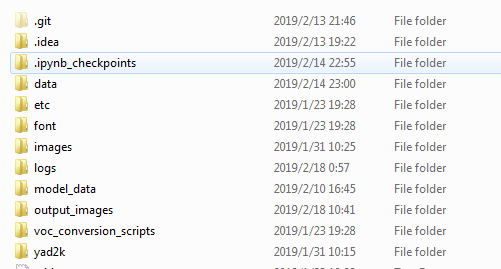data 中有 underwater_classes.txt 和 underwater_data.npz 文件。

## 探索数据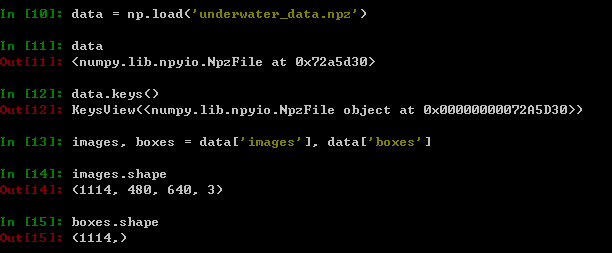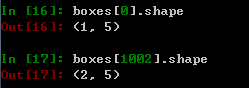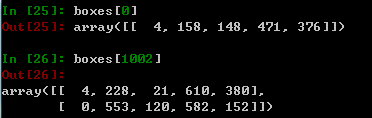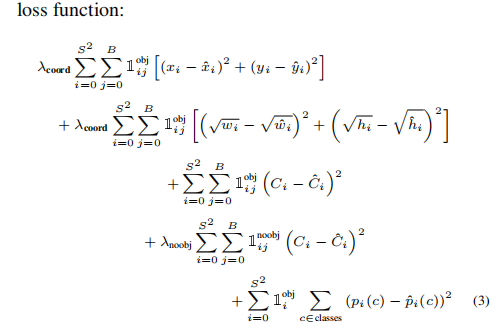# 调整数据格式

import os

import matplotlib.pyplot as plt
import numpy as np
import PIL
import tensorflow as tf
from keras import backend as K
from keras.layers import Input, Lambda, Conv2D
from keras.callbacks import TensorBoard, ModelCheckpoint, EarlyStopping



def __process_data(self, images, boxes):

images = [PIL.Image.fromarray(i) for i in images]
#保存图片原大小
orig_size = np.array([images.width, images.height])
orig_size = np.expand_dims(orig_size, axis=0)

processed_images = [i.resize((416, 416), PIL.Image.BICUBIC) for i in images]
processed_images = [np.array(image, dtype=np.float) for image in processed_images]
processed_images = [image/255. for image in processed_images]

# Get box parameters as x_center, y_center, box_width, box_height, class.
boxes_xy = [0.5 * (box[:, 3:5] + box[:, 1:3]) for box in boxes]
boxes_wh = [box[:, 3:5] - box[:, 1:3] for box in boxes]
boxes_xy = [boxxy / orig_size for boxxy in boxes_xy]
boxes_wh = [boxwh / orig_size for boxwh in boxes_wh]
boxes = [np.concatenate((boxes_xy[i], boxes_wh[i], box[:, 0:1]), axis=1) for i, box in enumerate(boxes)]

# 由于每个box的shape不一样， 为方便调整为一样的 shape
for i, boxz in enumerate(boxes):
if boxz.shape  < self.max_boxes:
zero_padding = np.zeros( (self.max_boxes-boxz.shape, 5), dtype=np.float32)

return np.array(processed_images), np.array(boxes)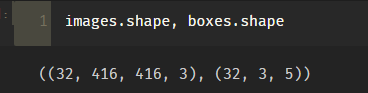def __get_detector_mask(self, boxes, anchors):

matching_true_boxes = []
for i, box in enumerate(boxes):
t_detectors_mask, t_matching_true_boxes = self.__preprocess_true_boxes(box, anchors, [416, 416])
matching_true_boxes.append(t_matching_true_boxes)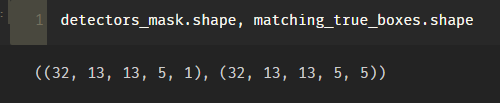__preprocess_true_boxes() 是将 box 和 anchor 以及 grid 相关联的方法， 即将对象分配到对应 grid 和 anchor 中。matching_true_boxes 就是分配完成后的结果， shape的解读为 ( x, y, w, h, class)。 至于 detectors_mask 则是计算在 grid 和 anchor 中是否出现了对象， 用于方便计算目标函数。 __preprocess_true_boxes() 的功能已经解读完毕，具体实现如下（由于较长， 在确定理解__get_detector_mask 的作用时， 可以暂时跳过这个详细部分， 直接进入一下部分）：

    def __preprocess_true_boxes(self, true_boxes, anchors, image_size):

height, width = image_size
num_anchors = len(anchors)
# Downsampling factor of 5x 2-stride max_pools == 32.
# TODO: Remove hardcoding of downscaling calculations.
assert height % 32 == 0, 'Image sizes in YOLO_v2 must be multiples of 32.'
assert width % 32 == 0, 'Image sizes in YOLO_v2 must be multiples of 32.'
conv_height = height // 32
conv_width = width // 32
num_box_params = true_boxes.shape
(conv_height, conv_width, num_anchors, 1), dtype=np.float32)
matching_true_boxes = np.zeros(
(conv_height, conv_width, num_anchors, num_box_params),
dtype=np.float32)

for box in true_boxes:
# scale box to convolutional feature spatial dimensions
box_class = box[4:5]
box = box[0:4] * np.array(
[conv_width, conv_height, conv_width, conv_height])
i = np.floor(box).astype('int')
j = np.floor(box).astype('int')
best_iou = 0
best_anchor = 0
for k, anchor in enumerate(anchors):
# Find IOU between box shifted to origin and anchor box.
box_maxes = box[2:4] / 2.
box_mins = -box_maxes
anchor_maxes = (anchor / 2.)
anchor_mins = -anchor_maxes

intersect_mins = np.maximum(box_mins, anchor_mins)
intersect_maxes = np.minimum(box_maxes, anchor_maxes)
intersect_wh = np.maximum(intersect_maxes - intersect_mins, 0.)
intersect_area = intersect_wh * intersect_wh
box_area = box * box
anchor_area = anchor * anchor
iou = intersect_area / (box_area + anchor_area - intersect_area)
if iou > best_iou:
best_iou = iou
best_anchor = k

if best_iou > 0:
[
box - j, box - i,
np.log(box / anchors[best_anchor]),
np.log(box / anchors[best_anchor]), box_class
],
dtype=np.float32)



# 创建模型

def create_model(anchors, class_names, load_pretrained=True, freeze_body=True):

detectors_mask_shape = (13, 13, 5, 1)
matching_boxes_shape = (13, 13, 5, 5)

# Create model input layers.
image_input = Input(shape=(416, 416, 3))
boxes_input = Input(shape=(3, 5))
matching_boxes_input = Input(shape=matching_boxes_shape)

# Create model body.
"""
中可以看到，  也有对应的 Yolo.cfg 参考。
跟 darkent 中 https://github.com/pjreddie/darknet/blob/master/cfg/yolov2.cfg 一致，
是新的网络结构
"""
yolo_model = yolo_body(image_input, len(anchors), len(class_names))
topless_yolo = Model(yolo_model.input, yolo_model.layers[-2].output)

"""
加载 pre-train-model （yolo.h5） 这里为了不覆盖而重新保存到 yolo_topless.h5 中
"""
# Save topless yolo:
topless_yolo_path = os.path.join('model_data', 'yolo_topless.h5')
if not os.path.exists(topless_yolo_path):
print("CREATING TOPLESS WEIGHTS FILE")
yolo_path = os.path.join('model_data', 'yolo.h5')
model_body = Model(model_body.inputs, model_body.layers[-2].output)
model_body.save_weights(topless_yolo_path)

if freeze_body:
for layer in topless_yolo.layers:
layer.trainable = False

# 创建最后一个输出层并创建一个完成的 Yolo_model
final_layer = Conv2D(len(anchors)*(5+len(class_names)), (1, 1), activation='linear')(topless_yolo.output)

model_body = Model(image_input, final_layer)

"""
这里其实是代替了 model.complie() 中给出了 Loss. 提前将目标函数写在这里， 然后传入 model 中。
再到 model.complie() 时传入 loss={'yolo_loss': lambda y_true, y_pred: y_pred}
这样做其实不太好， 有点混乱。 所以作者也弄了个 TODO: 期望用正式的 loss layer 来替代这个 Lambda
关于 Lambda 的用法，请到 keras 中查阅。  yolo_loss 一定要看 YOLO 论文中的公式， 因为这也是根据论文中的写出来的。

"""
# Place model loss on CPU to reduce GPU memory usage.
with tf.device('/cpu:0'):
# TODO: Replace Lambda with custom Keras layer for loss.
model_loss = Lambda(
yolo_loss,   # 传入 yolo_loss
output_shape=(1, ),  #期望输出的 shape ， 因为目标函数输出为一个常量， 故为(1, )
name='yolo_loss',
arguments={'anchors': anchors,        #传入参数， 这些参数是固定的， 生成 Lambda 对象时已经传入的
'num_classes': len(class_names)})([  # 运行时传入的参数， 对应到 yolo_loss 中的 args
model_body.output, boxes_input,
])

model = Model([model_body.input, boxes_input, detectors_mask_input,matching_boxes_input], model_loss)

return model_body, model


# 训练 Model

def train(model, class_names, anchors, image_data, boxes, detectors_mask, matching_true_boxes, validation_split=0.1):

"""
这里便是将 create_model 中的 yolo_loss 作为目标函数的方法， 这种用法的确很奇怪， 容易让人误解。
"""
model.compile(
'yolo_loss': lambda y_true, y_pred: y_pred
})  # This is a hack to use the custom loss function in the last layer.

logging = TensorBoard()
"""
训练 CNN 是十分耗时的事情， 所以这里会在每迭代完一次之后就保存当前训练的 model。
值得注意得是这里保存的模型为 traned____.h5 只是简单的加载了 create_model 中所谓的 pre-train-model
即 yolo_topless 。训练完后也没有更新 yolo_topless。 所以下次再训练时也是重头开始的， 部分代码可以自己微调。
"""
checkpoint = ModelCheckpoint("trained_stage_3_best.h5", monitor='val_loss',
save_weights_only=True, save_best_only=True)
early_stopping = EarlyStopping(monitor='val_loss', min_delta=0, patience=15, verbose=1, mode='auto')

np.zeros(len(image_data)),
validation_split=validation_split,
batch_size=32,
epochs=5,
callbacks=[logging])
model.save_weights('trained_stage_1.h5')

model_body, model = create_model(anchors, class_names, load_pretrained=False, freeze_body=False)

model.compile(
'yolo_loss': lambda y_true, y_pred: y_pred
})  # This is a hack to use the custom loss function in the last layer.

np.zeros(len(image_data)),
validation_split=0.1,
batch_size=8,
epochs=30,
callbacks=[logging])

model.save_weights('trained_stage_2.h5')

np.zeros(len(image_data)),
validation_split=0.1,
batch_size=8,
epochs=30,
callbacks=[logging, checkpoint, early_stopping])

model.save_weights('trained_stage_3.h5')



# 总结

yolo-v1-tensorflow ，这个网络的实现是跟YOLO 论文中的一模一样的， 同样理解此文章后可以自行研究这个版本。
yolo-v1-tensorflow，跟上面的基本一样，基本就是翻译过一遍注释。
darknet，一切的根源。

YOLO_learn